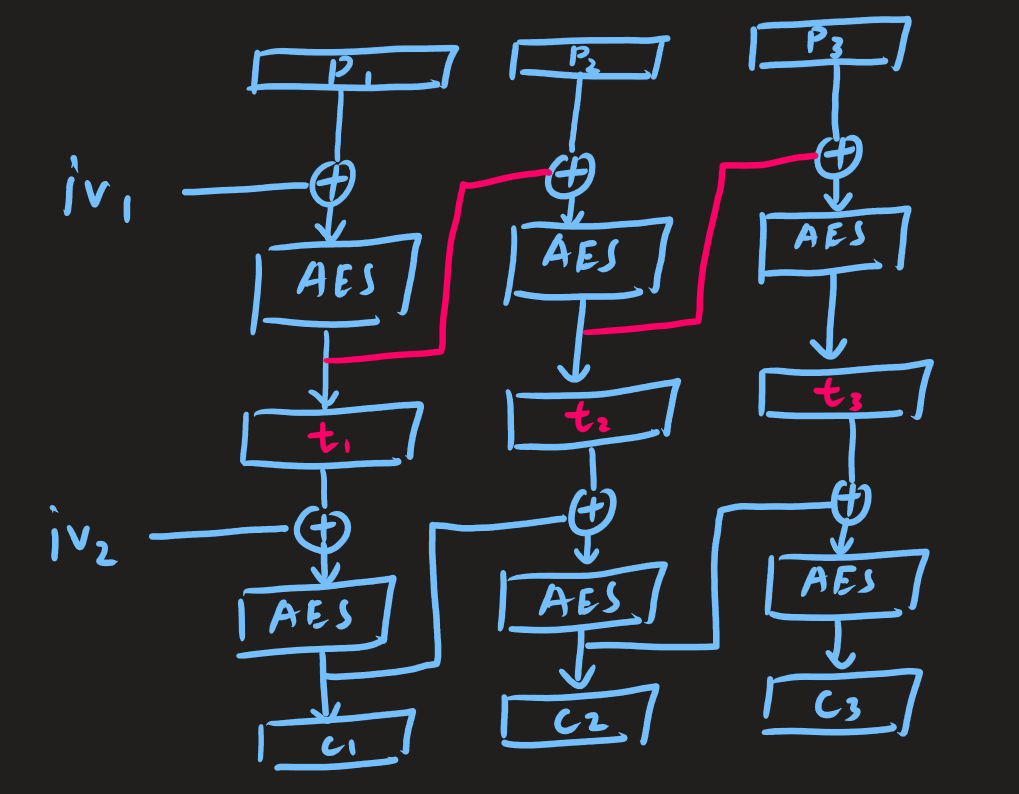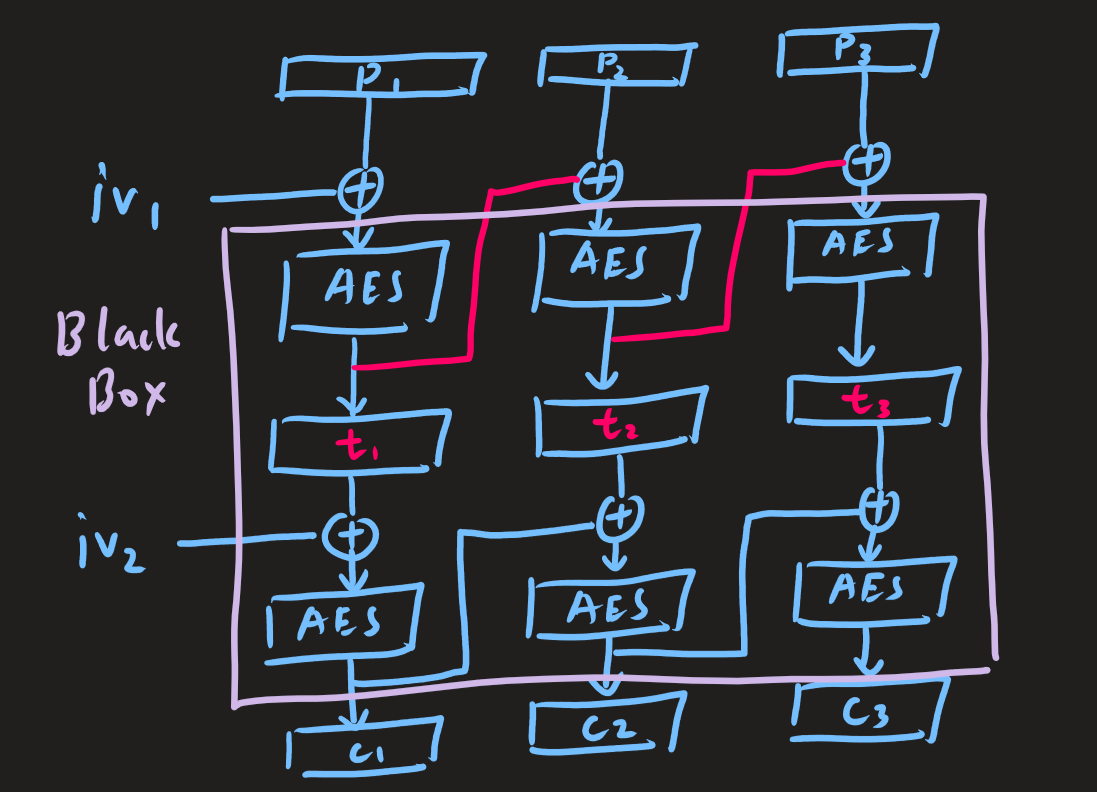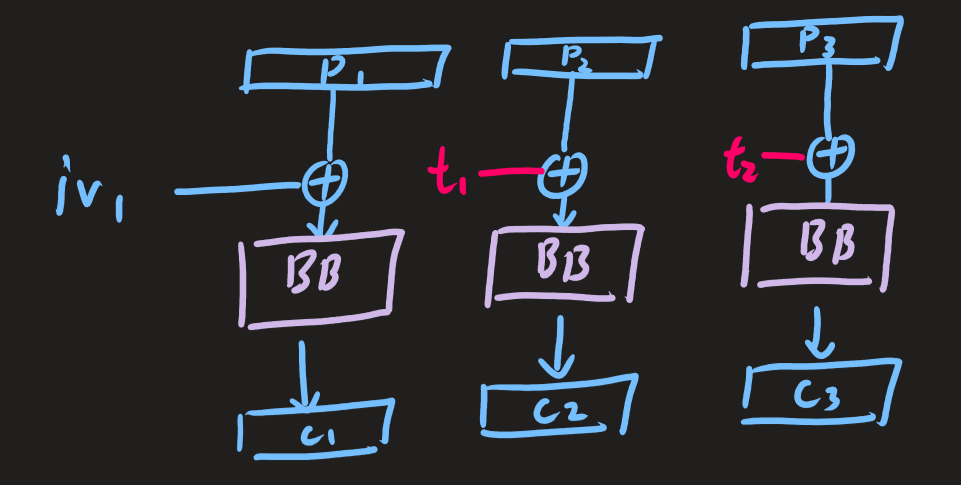CBCBC

This is the second crypto challenge from ASCS 2021. It tried to use double AES CBC to protect against padding oracle attack.

Statement

Wow, free flags! But encrypted with CBC… twice?

Observation

The objective of the challenge is to get the hidden_username.

We are provided both decryption and encryption (partially) oracle.

We are able to inject any string to the username section of the json data. The oracle will then spit out the encrypted text.

The decryption oracle will let us know if there are any padding error in the decrypted data.

Solution

The usual padding oracle attack in AES CBC does not require an encryption oracle. But it is not the case if AES CBC is used twice.

Here I draw the diagram for the encryption scheme.$$iv_{1}, iv_{2}, c_{i}$$ is known

Because padding oracle attack is based only on the chaining bytes and the output of each block, we can view the whole system as an blackbox.If $$t_{i}$$ is known, then it’s possible to find $$p_{i+1}$$ with padding oracle attack.

Note that $$t_{i}$$ and $$p_{i+1}$$ can swap places because of the xor property.

If $$p_{i+1}$$ is known, then it’s possible to find $$t_{i}$$ with padding oracle attack.

So the whole idea is to encrypt a known text using the encryption oracle provided.

We use padding oracle attack to recover $$t_{i}$$ and recover the hidden username afterwards.

Below is the python code solution for the challenge.

from pwn import *
import base64

r = remote('167.99.77.49', 52171)

r.sendline('1')
r.recvuntil('token: \n')
res = r.recvline()
c = base64.b64decode(res)

newiv = [0 for _ in range(16)]
ori = [0 for _ in range(16)]
pt = [0 for _ in range(16)]
for byte in range(16):
for j in range(16 - byte, 16):
newiv[j] = ori[j] ^ (byte + 1)
for j in range(2 ** 8):
newiv[15 - byte] = j
payload = bytes(newiv) + c[32 + 16 * 0:64 + 16 * 0]
r.sendline('2')
r.sendline('hello')
res = r.recvline()
if (b'Your' in res):
ori[15 - byte] = j ^ (byte + 1)
pt[15 - byte] = ori[15 - byte] ^ ord('a')
print(bytes(pt))
break

t1 = bytes(pt)

r.sendline('1')
r.sendline()
r.recvuntil('token: \n')
res = r.recvline()
c = base64.b64decode(res)

ans = b''

newiv = [0 for _ in range(16)]
ori = [0 for _ in range(16)]
pt = [0 for _ in range(16)]
for byte in range(16):
for j in range(16 - byte, 16):
newiv[j] = ori[j] ^ (byte +1)
for j in range(2 ** 8):
newiv[15 - byte] = j
payload = bytes(newiv) + c[32 + 16 * 0:64 + 16 * 0]
r.sendline('2')
r.sendline('hello')